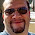## Tuesday, May 17, 2016

### HP Prime: Algebraic CAS Commands

HP Prime:  Algebraic CAS Commands

On today’s entry I am going discuss several CAS (Computer Algebraic System) commands: factor, expand, collect, and subst (substitute).   These four commands are common to calculators, mathematical software, and mathematical apps that have CAS commands.

If you have not heard about CAS before, CAS allows the user to operate on expressions with indefinite (undefined) variables.  CAS commands commonly manipulate algebraic expressions, calculate derivatives of functions, and calculate indefinite integrals.    My first experience with CAS commands was with the (original) TI-89 almost a decade and half ago.  (Wow, I’m getting old).  Let’s get started.

On the HP Prime, you can enter CAS mode by pressing the [CAS] button. In CAS mode, the variables are primarily lower case.  Single letter uppercase variables, along with θ, are always numeric.

Factor

Two type of factoring functions:  factor and ifactors.

factor:  [Toolbox], (CAS), 1. Algebra, 4. Factor

The command factor works with polynomials and rational expressions.

Examples:
factor(x^2 + 6*x + 5) returns (x + 1)*(x + 5)
factor(x^2 + x – 4) returns (x + (1 - √17) / 2) * (x + (1 + √17) / 2)
factor(x^2 + 4*x + 4) returns (x + 2)^2

TIP:  If you are not sure whether implied multiplication will be accepted, insert the multiply operators (*) in the expression.  It is always a safe bet.

ifactors:  [Toolbox], (CAS), 5.  Integer, 2. Factors

Unlike factor, ifactors works on factoring integers into their prime factorizations.  If the integer is prime, the integer itself is returned.

Examples:
ifactors(55) returns 5 * 11
ifactors(2162) returns 2 * 23 * 47
ifactors(1367) returns 1367   (1367 is prime)

Expand

There are three expand commands we’ll cover:  expand, powexpand, and texpand.  Which command that you use determines what time of expression to be expanded.

expand:  [Toolbox], (CAS), 1.  Algebra, 4.  Factor

The command expand works on polynomials and rational functions.  It can be thought of that expand is the opposite of factor.

Examples:
expand( (x - 1)^3 ) returns x^3 – 3*x^2 + 3*x - 1
expand( (x + 1)^2 * (x^2 – 3) ) returns x^4 + 2*x^3 – 2*x^2 – 6*x – 3

powexpand:  [Toolbox], (CAS), 4.  Rewrite, 2.  powexpand

The command powexpand involves expressions with exponents.

powexpand( x^(2 + a) ) returns x^2 * x^a

Sometimes you have to combine one or more CAS commands to get what you want.

powexpand( x^((a + 1) * (a + 5)) ) returns x^((a + 1) * (a + 5)).

This is not a result I would want.  Let’s tackle this another way:

expand( (a + 1)*(a + 5) ) returns a^2 + 6*a + 5, then
powexpand( x^(a^2 + 6*a + 5) ) returns x^a^2 * (x^a)^6 * x^5

texpand:  [Toolbox], (CAS), 4. Rewrite, 3. Texapnd

The command texpand is part of the expand family, this time texpand works with transcendental functions (sin, cos, tan, ln, exp).

texpand( sin(2*x) ) returns 2 * cos(x) * sin(x)
texpand( cos (2*x + 1) ) returns (2 * cos(x)^2 – 1) * cos(1) – 2 * cos(x) * sin(x) * sin(1)
texpand( e^(3*x) + cos(3*x) ) returns e^x^3 + 4 * cos(x)^3 – 3 * cos(x)

Collect

collect:  [Toolbox], (CAS), 1.  Algebra, 2.  Collect

This commands collects like terms in a polynomial, and when applicable factorizes the expressions.  The variable to collect around may be specified.

Examples:

collect( (x + 1)^2 + x^2 + 2*x ) returns 2*x^2 + 4*x + 1

collect( (x + a + 2*(x + a) ) returns 3 * (a + x)
collect( (x + a + 2*(x + a), a ) returns 3*a + 3*x
collect( (x + a + 2*(x + a), x ) returns 3*a + 3*x

collect( (x^2 + a^2 + 2*(x + a)^2 ) returns 4*a*x + 3*a^2 + 3*x^2
collect( (x^2 + a^2 + 2*(x + a)^2, a ) returns a * (3*a + 4*x) + 3*x^2
collect( (x^2 + a^2 + 2*(x + a)^2, x ) returns x * (4*a + 3*x) + 3*a^2

Substitute

This does exactly what it is says:  substitute.
Syntax:  subst(expression, var = value/expr)

subst:  [Toolbox], (CAS), 1. Algebra, 6. Substitute

Examples:

subst( x^4 + 2*x^2 – x, x = √a) returns a^2 + 2*a - √a

Here are two examples where an additional CAS command is needed to simplify:

subst( cos(2*x), x = asin(a) ) returns cos( 2* asin(a) )
texpand(cos( 2* asin(a) ) returns 2*(1 – a^2) – 1

subst( 2*x^2 – x, x = a + 3 ) returns 2*(a + 3)^2 – a – 3
expand(2*(a + 3)^2 – a – 3 ) returns 2*a^2 + 11*a + 15

There are four common CAS commands, I hope you find this helpful.

Eddie

This blog is property of Edward Shore, 2016.

1.Thanks Eddie! As ALWAYS, your blog is awesome and I look forward to reading every entry you create.

1.Thank you Bill! Appreciate the love.

Eddie

2.Thanks Eddie, very useful :-)

### TI-84 Plus CE: Simple Elliptic Curves Determined by Two Points

TI-84 Plus CE:  Simple Elliptic Curves Determined by Two Points Introduction The program ECURVE determines the coefficients of a simple...